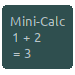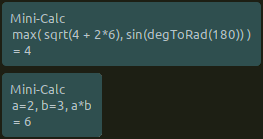# Mini-Calc Desklet

UUID: calc@ptandler
Last edited:
2 months ago 2021-09-21, 06:59Very simple and minimalistic but yet powerful calculator that evaluates JavaScript expressions.

# Mini-Calculator Desklet based on JavaScript Expressions

Very simple and minimalistic but yet quite powerful calculator that evaluates JavaScript expressions. The complete power of JS directly at your hand! ;-)

## Keyboard Input

Keyboard input is available after the desklet was raised to top by the defined shortcut (default SUPER+C, can be changed in the desklet settings).

## Features and Example Expressions

• `1+2` = `3`
• `(1 + 2) * (3 + 4)` = `21`
• All functions defined in the JavaScript `Math` object are included using the `with` statement (knowing that is not generally recommended using `with` in JS, but I think here it's kind of convenient).
• `min(1,2)` = `1`
• `max(1,2)` = `2`
• `sqrt(16)` = `4`
• `abs(-1)` = `1`
• The trigonometric functions `sin(), cos(), tan(), asin(), acos(), atan(), and atan2()` expect (and return) angles in radians.
• Helper functions: `degToRad(180)` = approx. `3.14`, `radToDeg(PI)` = `180`
• `random()` returns a pseudo-random number between 0 and 1.
• Euler's constant: `E` = approx. `2.718`
• `PI` = approx. `3.14159`
• You can define variables; this expression will return the assigned value. The variables will keep their value until the desklet is restarted:
• `a=2` = `2`
• You can use the comma `,` operator to evaluate several expressions and return just the value of the last one. Can be used to define variables:
• `a=2, b=3, a*b`
• For conditional expression you can use the JS `? :` operator, e.g. `1 < 2 ? "that's true" : "no, wrong"`
• You can define functions:
• `fib = (n) => n <= 0 ? 0 : (n <= 1 ? 1 : (fib(n - 1) + fib(n - 2))), fib(7)` = `13`
• You can use all builtin JavaScript functions and global objects (available in GJS / CJS), such as `JSON.stringify`

## ToDo: Ideas for Further Development

• add expression & result history as dropdown with scrollbar (on pressing "enter" + clear text input)
• add action "copy value to clipboard" including keyboard shortcut; also allow copying the expression; and of course allow copying all past expressions and results from the history
• add icon to open message box for documentation and introduction that explains some JS expression syntax (e.g. the examples above)
• add option to turn off to use `JSON.stringify()` to display results